Home | | Engineering Mathematics I | Multiple Integrals

# Multiple Integrals

Introduction, Evaluation Of Double Intgrals, Problems Based On Double Integration In Cartesian Coordinates, Double Integration In Polar Coordinates, Change The Order Of Integration, Problems Based On Area As A Double Integral, Evaluation Of Triple Integrals.

MULTIPLE INTEGRALS

Introduction

Evaluation Of Double Intgrals

Problems Based On Double Integration In Cartesian Coordinates

Double Integration In Polar Coordinates

Change The Order Of Integration

Problems Based On Area As A Double Integral

Evaluation Of Triple Integrals

INTRODUCTION: When a unction f(x) is integrated with respect to x between the limits a and b, get the definite integral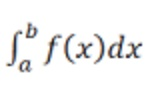If the integrand is a function f(x,y) and if it is integrated with respect to x and y repeatedly between the limits x0 and x1 (or x) between the limits y0 and y1 (or y).

we get a double integral that is denoted by the symbolExtending the concept of double integral one step further, we get the tripe integral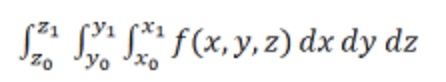EVALUATION OF DOUBLE INTGRALS

Before starting on double integrals let’s do a quick review of the definition of a definite integrals for functions of single variables. First, when working with theWe think of x's as comimg from the interval a<x<b. For these integrals we can say that we are integrating over the interval a<x<b. Note that this does assume that a<b, however, if we have b<a then we can just use interval b<x<a.

Now, when we derived the definition of the definite integral we first thought of this as an area problem. We first asked what the area under the curve was and to do this we broke up the interval a<x<b into n subintervals of width Del(x) ∆x and choose a point, x1 from each as shown below.Each of the rectangles has height of f(x1) and we could then use the area of each of these rectangles to approximate the area as follows.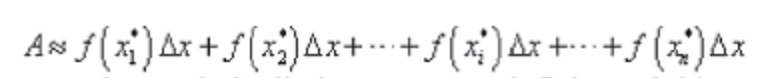To get the exact area we then took the limit as n goes to iniinity and this was also the definition of the definite integral.we first integrate f(x,y) with respect to x partially, treating y as a constant temporarily, between x0 and x1. The resulting function got after the inner integration and substitution of limits will be a function of y. Then we integrate this function of y with respect to y between the limits y0 and y1 as usual.

PROBLEMS BASED ON DOUBLE INTEGRATION IN CARTESIAN COORDINATES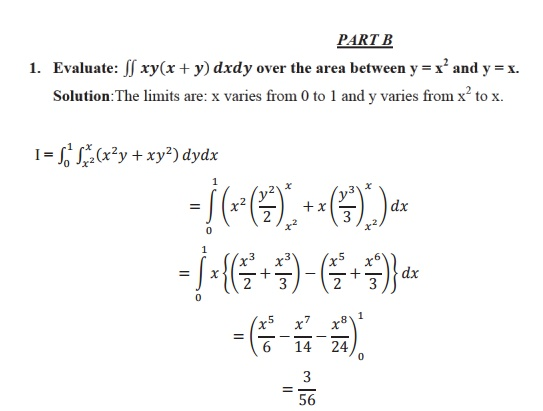DOUBLE INTEGRATION IN POLAR COORDINATES:, we first integrate w.r.to r between the limits r1 and r2. Keeping θ1 is fixed and the resulting expression is integrated w.r.to θ from θ1 to θ2.

In this integral this r1 and r2 are functions of θ and θ1, θ2 are constants.CHANGE THE ORDER OF INTEGRATION:

The double integralwhen the order of integration is changed. This process of converting a given double integral into its equivalent double integral by changing the order of integration is often called change of order of integration. To effect the change of order of integration, the region of integration is identified first, a rough sketch of the region is drawn and then the new limits are fixed.PROBLEMS BASED ON AREA AS A DOUBLE INTEGRAL:

Area of the region R in Cartesian form is given by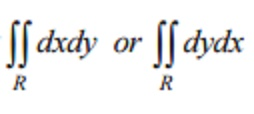Area of the region R in polar form is given by5. Find the area inside the circle r=asinθ but lying outside the cardiod r=a(1-cosθ)

Solution: Given curves are r=asinθ and r =a(1-cosθ)

The curves intersect where a sin θ = a (1-cosθ)EVALUATION OF TRIPLE INTEGRALS

To evalute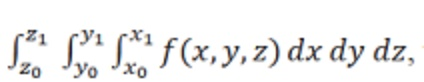,

we first integrate f(x,y,z) with respect to x, treating y and x as constants temporarily. The limits x0 and x1 may be constants or unctions of y and z. Then we perform the middle integration with respect to y, treating function got after  the middle integrtion may be a unction o z only. Finally we perform the  outermost the outermost integration with respect to z between the constant limits z0 and z1.

The following sketch shows the relationship between the Cartesian and spherical coordinate systems.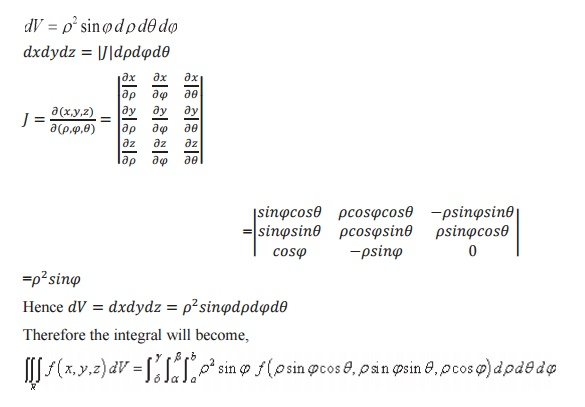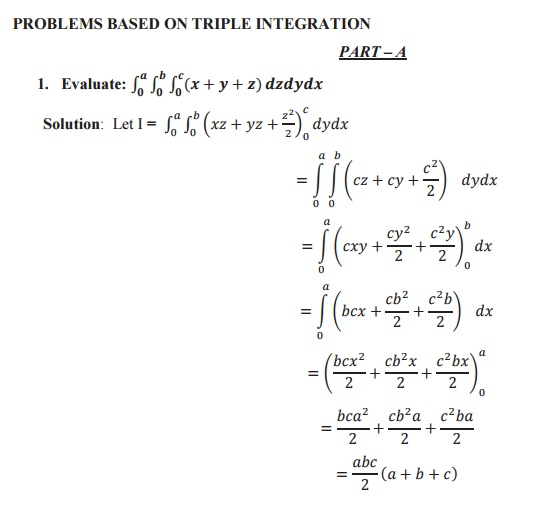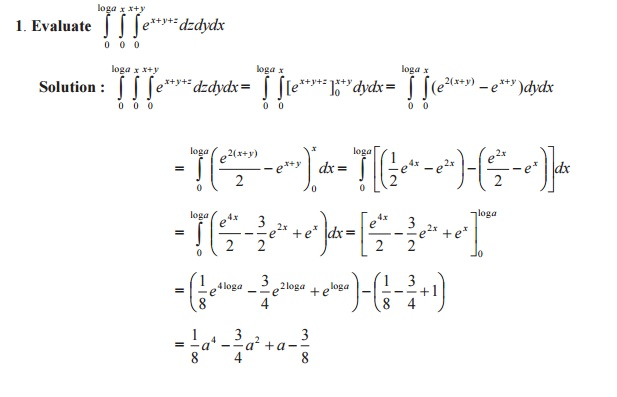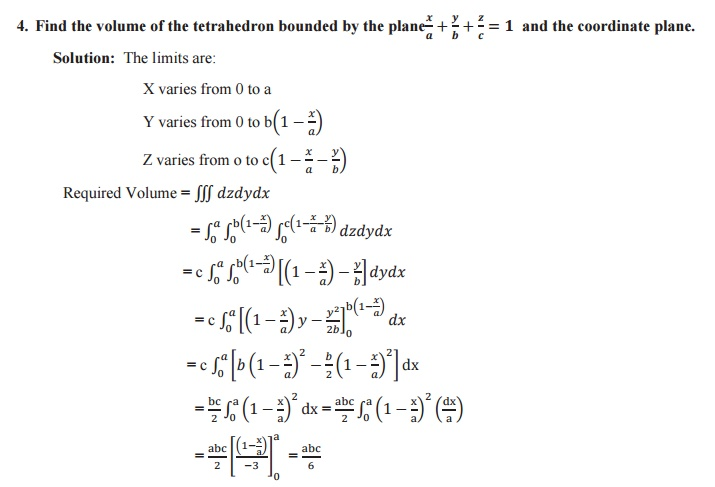Study Material, Lecturing Notes, Assignment, Reference, Wiki description explanation, brief detail
Mathematics : Multiple Integrals : Multiple Integrals |

Related Topics